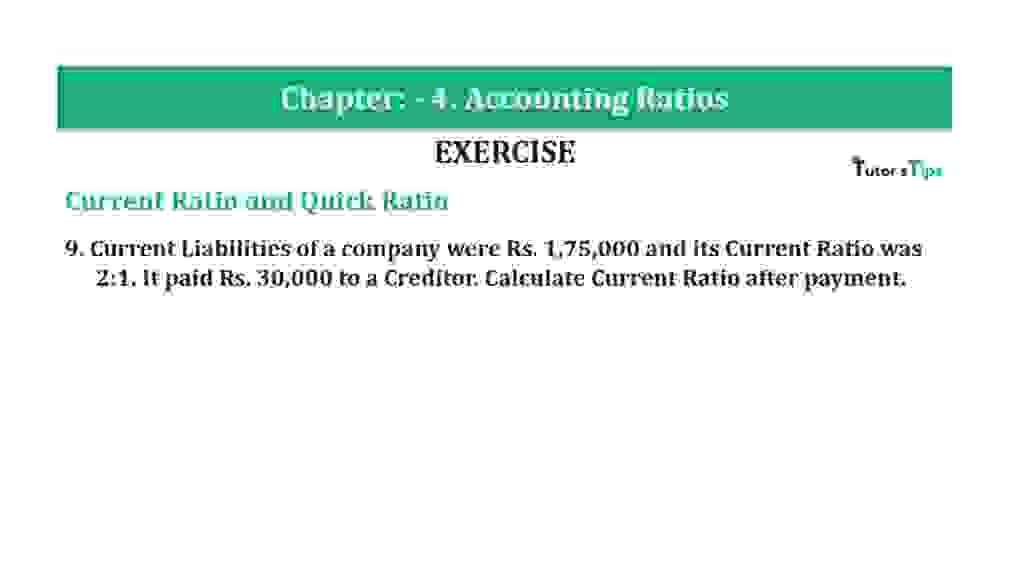# Question 9 Chapter 4 of +2-B – T.S. Grewal 12 ClassQuestion 9 Chapter 4 of +2-B

Current Ratio and Quick Ratio

9. Current Liabilities of a company were Rs. 1,75,000 and its Current Ratio was 2:1. It paid Rs. 30,000 to a Creditor. Calculate Current Ratio after payment. Ratio after the purchase.

### The solution of Question 9 Chapter 4 of +2-B: –

 Current Ratio =2: 1
 Current Liabilities =Rs. 1,75,000

Payment of Rs.30,000 to a Creditor will have two effects:

(i) Decrease in Cash by Rs.30,000

 Current Assets= Rs. 3,50,000 – Rs. 30,000
 Current Assets= =Rs. 3,20,000

(ii) Decrease in Creditors by Rs.30,000

 Current Liabilities= Rs. 1,75,000 – Rs. 30,000
 Current Liabilities= Rs. 1,45,000

 Current Ratio= Current Assets Current Liabilities
 Current Ratio= 3,20,000 1,45,000

Current Ratio=2.21 : 1

Balance Sheet: Meaning, Format & Examples

Thanks, Please Like and share with your friends

Comment if you have any question.

Also, Check out the solved question of previous Chapters: –

### T.S. Grewal’s Double Entry Book Keeping (Vol. II: Accounting for Companies)T.S. Grewal’s Analysis of Financial Statements